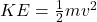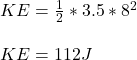## A 3.5kg bowling ball rolls down the alley at 8m/s. Calculate the kinetic energy that it hits the pins with how many jules

Question

A 3.5kg bowling ball rolls down the alley at 8m/s. Calculate the kinetic energy that it hits the pins with how many jules

in progress 0
6 months 2021-08-22T14:48:35+00:00 1 Answers 2 views 0

## Answers ( )

112 J

Explanation:

The kinetic energy of a body is given as:where m = mass

v = velocity

The mass of the ball is 3.5 kg.

The velocity of the ball is 8 m/s.

The kinetic energy of the ball is therefore: